• 贝叶斯判别分析，用于降维分类，包含多个程序，亲测可以有实例
• 今阅读“Data Science and machine learning” Chapter 7，对西瓜书描述的线性判别分析有了新的理解，在此分享。 Reference : Rocklv J , Gayle A A . Data Science and Machine Learning: Mathematical and ...
今阅读“Data Science and machine learning” Chapter 7，对西瓜书描述的线性判别分析有了新的理解，在此分享。Reference : Rocklv J , Gayle A A . Data Science and Machine Learning: Mathematical and Statistical Methods. D.P. Kroese, Z.I. Botev, T. Taimre, and R. Vaisman[J]. International Journal of Epidemiology, 2020.
展开全文算法 机器学习
• 线性回归线性分类硬输出-感知机硬输出-线性判别分析软输出-判别式：逻辑回归软输出-生成式：高斯判别分析软输出-生成式：朴素贝叶斯 线性分类 从线性回归到线性分类，通过激活函数或者降维来实现。 线性回归函数f...


线性回归
线性分类硬输出-感知机硬输出-线性判别分析软输出-判别式：逻辑回归软输出-生成式：高斯判别分析软输出-生成式：朴素贝叶斯

线性分类
从线性回归到线性分类，通过激活函数或者降维来实现。
线性回归函数

f

(

w

,

b

)

=

w

T

x

+

b

f(w,b)=w^Tx+b

经过激活函数作用

y

=

f

(

w

T

x

+

b

)

y=f(w^Tx+b)

作用后变成硬分类问题

y

ϵ

{

0

,

1

}

y\epsilon \left \{0,1 \right \}

或者变成软分类问题

y

ϵ

[

0

,

1

]

,

y\epsilon [0,1],

函数

f

f

就称为激活函数，

f

−

1

f^{-1}

称为

l

i

n

k

f

u

n

c

t

i

o

n

,

硬分类代表模型：感知机，线性判别分析 软分类代表模型：高斯判别分析(生成式模型)，逻辑回归(判别式模型)
硬输出-感知机
样本集：

{

(

x

i

,

y

i

)

}

i

=

1

N

\left \{ (x_i,y_i)\right \}_{i=1} ^N

感知机思想：错误驱动。给定一个初始的分类平面

w

T

x

，

w^Tx，

再一步步移动这个平面使得被错误分类的点越来越少。被错误分类的集合用D表示 模型：

f

(

x

)

=

s

i

g

n

(

w

T

x

)

,

x

ϵ

R

P

,

w

ϵ

R

P

,

f(x)=sign(w^Tx),x\epsilon R^P,w\epsilon R^P,

其中当

w

T

x

≥

0

,

s

i

g

n

(

w

T

x

)

=

1

;

w

T

x

&lt;

0

,

s

i

g

n

(

w

T

x

)

=

0

w^Tx \geq 0,sign(w^Tx)=1;w^Tx &lt; 0,sign(w^Tx)=0

策略定义为被错误分类的点的个数 策略：

l

o

s

s

f

u

n

c

t

i

o

n

:

L

(

w

)

=

∑

i

=

1

N

I

{

y

i

w

T

x

i

&lt;

0

}

,

loss function:L(w)=\sum_{i=1} ^N I\left \{ y_iw^Tx_i&lt;0 \right \},

也就是当

y

i

w

T

x

i

&lt;

0

y_iw^Tx_i&lt;0

时样本是被错误分类的。 通俗点理解就是

w

T

x

i

&gt;

0

,

y

i

=

1

→

y

i

w

T

x

i

&gt;

0

,

w^Tx_i&gt;0,y_i=1\rightarrow y_iw^Tx_i&gt;0,

分类正确，反之分类错误。然而

L

(

w

)

L(w)

是不可导的，那么这个

l

o

s

s

f

u

n

c

t

i

o

n

loss function

就需要进行改进。虽然

L

(

w

)

L(w)

是不可导的，但是

y

i

w

T

x

i

y_iw^Tx_i

是可导的。 因此改进之后的

l

o

s

s

f

u

n

t

i

o

n

loss funtion

是这样：

L

(

w

)

=

∑

x

i

ϵ

D

−

y

i

w

T

x

i

，

L(w)=\sum_{x_i\epsilon D}-y_iw^Tx_i，

对

w

w

求偏导：

▽

w

L

=

−

y

i

x

i

\bigtriangledown _wL=-y_ix_i

接下来算法只需用随机梯度下降法就能求出来了。 算法:SGD

w

(

t

+

1

)

←

w

t

+

λ

▽

w

L

w^{(t+1)}\leftarrow w^{t}+\lambda \bigtriangledown _wL

当然，上述的感知机算法都是基于数据线性可分为前提
硬输出-线性判别分析
样本集：

{

(

x

i

,

y

i

)

}

i

=

1

N

,

x

i

ϵ

R

p

,

y

i

ϵ

{

c

1

=

1

,

c

2

=

−

1

}

\left \{ (x_i,y_i)\right \}_{i=1} ^N,x_i\epsilon R^p,y_i\epsilon\left\{ c_1=1,c_2=-1 \right \}

x

c

1

=

{

x

i

∣

y

i

=

+

1

}

,

x

c

2

=

{

x

i

∣

y

i

=

−

1

}

x_{c_1}=\left \{x_i|y_i=+1 \right \},x_{c_2}=\left \{x_i|y_i=-1 \right \}

令集合

x

c

1

=

N

1

,

x

c

2

=

N

2

,

x_{c_1}=N_1,x_{c_2}=N_2,

所以

N

1

+

N

2

=

N

N_1+N_2=N

思想：类内小，类间大 如图所示：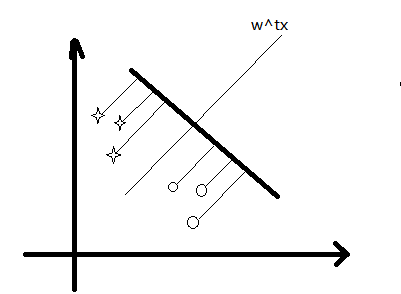同类的数据间隔尽量小(类内方差尽量小)，而不同类数据星星和圈圈之间的距离尽量大(类间大)。 我们投影的方向是

w

,

w,

也就是超平面对应的法向量。 点到平面的投影的线为

z

，

z

i

=

w

T

x

i

z，z_i=w^Tx_i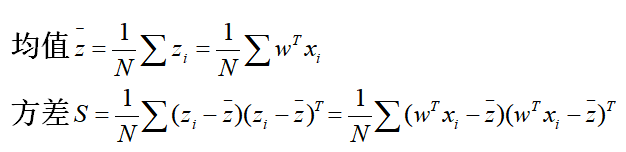所以不同类的均值可以表示出来：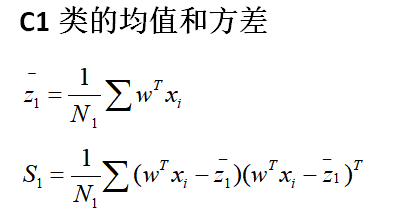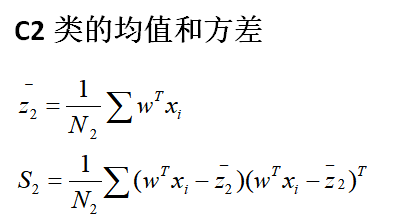那么类间的距离就可以用

(

z

ˉ

1

−

z

ˉ

2

)

2

(\bar z_1-\bar z_2)^2

表示； 类内的距离就用

S

1

+

S

2

S_1+S_2

表示。 那么为了达到我们的目的，也就是类内小，类间大，目标函数可以定义如下：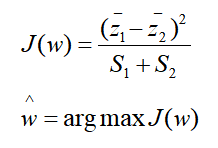令：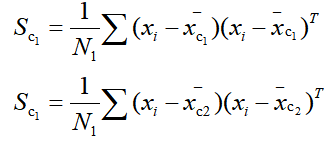化简我们的

J

(

w

)

J(w)

表达式，可得：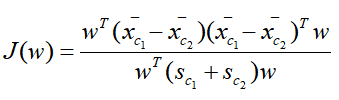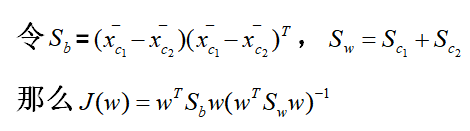这里的

S

b

S_b

就是类间方差，

S

w

S_w

是类内方差。 对w求偏导，令

∂

J

(

w

)

∂

w

=

0

，

\frac{\partial J(w)}{\partial w}=0，

求得：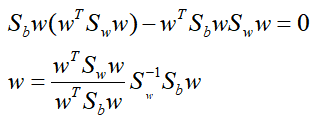由于我们只关心

w

w

的方向而不管其大小，那么

w

∝

S

w

−

1

(

x

ˉ

c

1

−

x

ˉ

c

2

)

w \propto S_w^{-1}(\bar x_{c_1}-\bar x_{c_2})

；如果

S

w

−

1

S_w^{-1}

是个对角矩阵或者单位矩阵，那么

w

∝

(

x

ˉ

c

1

−

x

ˉ

c

2

)

w \propto (\bar x_{c_1}-\bar x_{c_2})

软输出-判别式：逻辑回归
样本集：

{

(

x

i

,

y

i

)

}

i

=

1

N

,

x

i

ϵ

R

p

,

y

i

ϵ

{

0

,

1

}

\left \{ (x_i,y_i)\right \}_{i=1} ^N,x_i\epsilon R^p,y_i\epsilon\left\{ 0,1 \right \}

引入sigmoid function：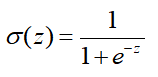其函数图像如下：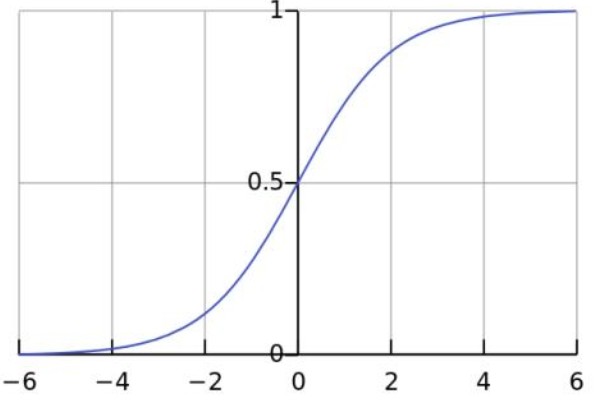代入有：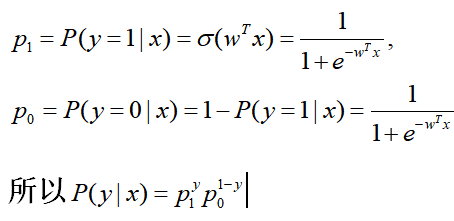接下来用极大似然估计求解

w

^

=

a

r

g

m

a

x

w

l

o

g

P

(

Y

∣

X

)

\hat{w}=\underset w {argmax}logP(Y|X)

就可以了。
软输出-生成式：高斯判别分析
样本集：

{

(

x

i

,

y

i

)

}

i

=

1

N

,

x

i

ϵ

R

p

,

y

i

ϵ

{

0

,

1

}

\left \{ (x_i,y_i)\right \}_{i=1} ^N,x_i\epsilon R^p,y_i\epsilon\left\{ 0,1 \right \}

我们知道对于概率判别模型，我们可以直接求y值进行分类；而概率生成模型则是通过比较y=0和y=1的大小进行判断，根据贝叶斯公式

P

(

y

∣

x

)

=

P

(

x

∣

y

)

P

(

y

)

P

(

x

)

P(y|x)=\frac{P(x|y)P(y)}{P(x)}

我们知道，概率生成模型和分母项

P

(

x

)

P(x)

无关，所以可以对联合概率建模

P

(

y

∣

x

)

∝

P

(

x

∣

y

)

P

(

y

)

，

P(y|x)\propto P(x|y)P(y)，

也就是后验=似然*先验。
模型：

y

^

=

a

r

g

m

a

x

y

ϵ

{

0

,

1

}

P

(

y

∣

x

)

=

a

r

g

m

a

x

y

P

(

y

)

P

(

x

∣

y

)

\hat y=\underset{y\epsilon\left \{0,1\right\}}{argmax}P(y|x)=\underset y {argmax}P(y)P(x|y)

那么y是服从伯努利分布

P

(

y

)

=

ϕ

y

(

1

−

ϕ

)

1

−

y

P(y)=\phi^y(1-\phi)^{1-y}

，
x服从于高斯分布,

x

∣

y

=

1

∼

N

(

μ

1

,

ε

)

y

x|y=1 \sim N(\mu_1,\varepsilon )^y

,

{

x

i

∣

y

i

=

1

,

i

=

1

,

.

.

.

,

N

}

\left \{x_i|y_i=1,i=1,...,N\right \}

,此集合作为

c

1

c_1

,集合内元素的总个数为

N

1

N_1

，对应的方差为

S

1

S_1

。

x

∣

y

=

0

∼

N

(

μ

2

,

ε

)

y

x|y=0 \sim N(\mu_2,\varepsilon )^y

,

{

x

i

∣

y

i

=

0

,

i

=

1

,

.

.

.

,

N

}

\left \{x_i|y_i=0,i=1,...,N\right \}

,集合作为

c

2

c_2

,集合内元素的总个数为

N

2

N_2

，对应的方差为

S

2

S_2

。

P

(

x

i

∣

y

i

)

=

N

(

μ

1

,

ε

)

y

∗

N

(

μ

2

,

ε

)

1

−

y

P(x_i|y_i)=N(\mu_1,\varepsilon )^y*N(\mu_2,\varepsilon )^{1-y}

对数似然就能写成如下公式：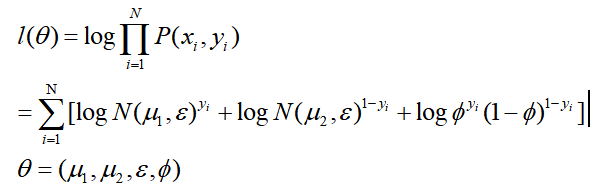求得

ϕ

^

=

N

1

N

,

\hat \phi=\frac{N_1}{N},

ε

^

=

1

N

(

N

1

S

!

+

N

2

S

2

)

\hat\varepsilon=\frac{1}{N}(N_1S_!+N_2S_2)

μ

^

1

=

∑

i

=

1

N

y

i

x

i

N

1

\hat\mu_1=\frac{\sum{i=1}{N}y_ix_i}{N_1}

μ

^

2

=

∑

i

=

1

N

y

i

x

i

N

2

\hat\mu_2=\frac{\sum{i=1}{N}y_ix_i}{N_2}

软输出-生成式：朴素贝叶斯
样本集：

{

(

x

i

,

y

i

)

}

i

=

1

N

,

x

i

ϵ

R

p

,

y

i

ϵ

{

0

,

1

}

\left \{ (x_i,y_i)\right \}_{i=1} ^N,x_i\epsilon R^p,y_i\epsilon\left\{ 0,1 \right \}

思想：朴素贝叶斯假设，也叫条件独立性假设。 朴素贝叶斯是最简单的概率图(有向图)模型。 条件独立性假设如图所示: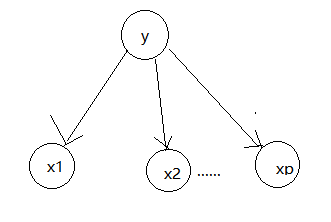也就是给定y的情况下，x1和x2相互独立。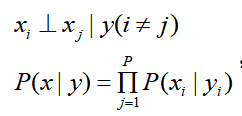做这个假设的目的就是为了简化运算，考虑高维情况。
朴素贝叶斯的目的也是给定x和y，判断其属于0还是1。 模型：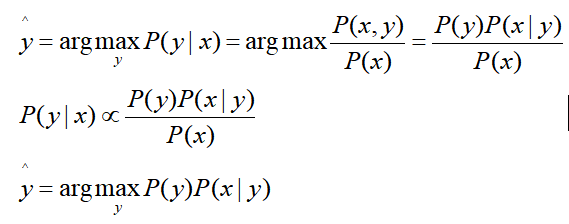对于P(y)，如果是二分类问题，其属于伯努利分布；如果是多分类问题，其属于categrial分布。 对于P(x|y),假定其遵循条件独立性假设。如果x是离散的，其属于categorical分布；如果x是连续的，其属于高斯分布。
求解可以直接用极大似然估计求解出来，在此就不做过多介绍了。
展开全文逻辑回归 感知机算法
• 单变量线性判别分析分类方法单变量线性判别分析贝叶斯方法进行分类单变量线性判别分析分类参数估计程序实现参考文献 单变量线性判别分析贝叶斯方法进行分类 线性判别分析贝叶斯分类方法的关系十分紧密。我们...


单变量线性判别分析分类方法
单变量线性判别分析用贝叶斯方法进行分类单变量线性判别分析分类参数估计程序实现参考文献

单变量线性判别分析
用贝叶斯方法进行分类
线性判别分析（linear discriminant analysis, LDA）与贝叶斯分类方法的关系十分紧密。我们先来看怎么用贝叶斯方法进行分类。
假设我们有数据集

{

(

X

1

,

Y

1

)

,

(

X

2

,

Y

2

)

,

⋯

,

}

\{(X_1, Y_1), (X_2, \, Y_2), \cdots, \}

。这里

X

X

是自变量，

Y

Y

是因变量，或称为测量量、要预测的变量。

X

i

X_i

可以是一个多维的向量。我们想要把测量到的点（观察点）

Y

i

Y_i

分为

K

K

类中的一类。我们假设每一类的先验概率为

π

k

\pi_k

，即如果我们没有其他信息，随机得抽取一个样本点，这个样本点属于第

k

k

类的概率就是

π

k

\pi_k

。我们用

f

k

(

x

)

=

P

(

X

=

x

∣

Y

=

k

)

\displaystyle f_k (x) = \mathbb{P} (X = x \vert Y = k)

来表示当观察点属于第

k

k

类时，自变量

X

X

的概率密度分布 （当

X

X

的取值为离散的时候，我们就用概率

P

(

X

=

x

∣

Y

=

k

)

\mathbb{P} (X = x \vert Y = k)

表示）。
于是，根据Bayes 公式，我们有

P

(

Y

=

k

∣

X

=

x

)

=

P

(

X

=

x

,

Y

=

k

)

P

(

X

=

x

)

=

P

(

X

=

x

,

Y

=

k

)

∑

ℓ

=

1

K

P

(

Y

=

ℓ

)

P

(

X

=

x

∣

Y

=

ℓ

)

=

π

k

f

k

(

x

)

∑

ℓ

=

1

K

π

ℓ

f

ℓ

(

x

)

\begin{aligned} \mathbb{P} (Y = k \vert X = x) &= \frac{\mathbb{P} (X = x, Y = k)}{\mathbb{P} (X = x)} \\ &= \frac{\mathbb{P} (X = x, Y = k)}{\sum_{\ell = 1}^K \mathbb{P}(Y = \ell) \mathbb{P}(X = x \vert Y = \ell)} \\ &= \frac{\pi_k f_k (x) }{\sum_{\ell = 1}^K \pi_{\ell} f_{\ell} (x)} \end{aligned}

所以，根据 Bayes 公式，如果我们能够计算出

π

k

f

k

(

x

)

∑

ℓ

=

1

K

π

ℓ

f

ℓ

(

x

)

\displaystyle \frac{\pi_k f_k (x) }{\sum_{\ell = 1}^K \pi_{\ell} f_{\ell} (x)}

的值，我们可以比较得出使得

π

k

f

k

(

x

)

∑

ℓ

=

1

K

π

ℓ

f

ℓ

(

x

)

\displaystyle \frac{\pi_k f_k (x) }{\sum_{\ell = 1}^K \pi_{\ell} f_{\ell} (x)}

的值最大的那个类

k

k

，从而将

X

=

x

X = x

这个数据分到第

k

k

类中。为了方便起见，我们用

p

k

(

x

)

p_k (x)

来表示

P

(

Y

=

k

∣

X

=

x

)

\mathbb{P} (Y = k \vert X = x)

。
如果我们有预先的知识能让我们决定先验分布

π

k

\pi_k

，我们可利用预先有的知识来确定

π

k

\pi_k

。否则，我们就用样本的频数来估计

π

k

\pi_k

。即

π

k

=

n

k

/

n

\displaystyle \pi_k = n_k / n

。这里

n

k

n_k

是属于第

k

k

类的样本点的数目。

n

n

是总共的样本数。
而线性判别分析，linear discriminant analysis (LDA) 就提供了一个估计

p

k

(

x

)

p_k (x)

的方法。下面我们就来介绍如何用 LDA 来进行贝叶斯公式的估计，从而对数据进行分类。
单变量线性判别分析分类
所谓单变量的线性判别分析，是指

X

X

只有一个变量，即

X

X

是一维的。我们假设

Y

Y

可以取三个不同的类别。为了估计

p

k

(

x

)

p_k (x)

，我们须要对

f

k

(

x

)

f_{k} (x)

有一个估计。这里线性判别分析做了一个假设，即假设

f

k

(

x

)

f_{k} (x)

服从一个正态分布。回忆上文，

f

k

(

x

)

\displaystyle f_k (x)

表示当观察点属于第

k

k

类时，自变量

X

X

的概率密度分布。根据这个假设，我们有

f

k

(

x

)

=

1

2

π

exp

⁡

(

−

(

x

−

μ

k

)

2

2

σ

k

2

)

f_k (x) = \frac{1}{\sqrt{2 \pi}} \exp \left( - \frac{(x - \mu_k)^2}{2 \sigma_k^2} \right)

有了对

f

k

(

x

)

f_k (x)

的估计，我们便可以计算

p

k

(

x

)

p_k (x)

。根据上面的公式，我们有

p

k

(

x

)

=

π

k

f

k

(

x

)

∑

ℓ

=

1

K

π

ℓ

f

ℓ

(

x

)

\displaystyle p_k (x) = \frac{\pi_k f_k (x) }{\sum_{\ell = 1}^K \pi_{\ell} f_{\ell} (x)}

。代入

f

k

(

x

)

f_{k} (x)

的表达式，我们有

p

k

(

x

)

=

π

k

1

2

π

exp

⁡

(

−

(

x

−

μ

k

)

2

2

σ

k

2

)

∑

ℓ

=

1

K

π

ℓ

1

2

π

exp

⁡

(

−

(

x

−

μ

ℓ

)

2

2

σ

ℓ

2

)

p_k (x) = \frac{\pi_k \frac{1}{\sqrt{2 \pi}} \exp \left( - \frac{(x - \mu_k)^2}{2 \sigma_k^2} \right)}{\sum_{\ell = 1}^K \pi_{\ell} \frac{1}{\sqrt{2 \pi}} \exp \left( - \frac{(x - \mu_\ell)^2}{2 \sigma_\ell^2} \right)}

如果我们再做一个假设，假设

σ

1

2

=

σ

2

2

=

⋯

=

σ

K

2

\displaystyle \sigma_1^2 = \sigma_2^2 = \cdots = \sigma_K^2

。即对于不同类别的数据，它们对应的

X

X

的分布的方差是相同的。那么我们就有

p

k

(

x

)

=

π

k

1

2

π

exp

⁡

(

−

(

x

−

μ

k

)

2

2

σ

2

)

∑

ℓ

=

1

K

π

ℓ

1

2

π

exp

⁡

(

−

(

x

−

μ

ℓ

)

2

2

σ

2

)

.

(1)

p_k (x) = \frac{\pi_k \frac{1}{\sqrt{2 \pi}} \exp \left( - \frac{(x - \mu_k)^2}{2 \sigma^2} \right)}{\sum_{\ell = 1}^K \pi_{\ell} \frac{1}{\sqrt{2 \pi}} \exp \left( - \frac{(x - \mu_\ell)^2}{2 \sigma^2} \right)}. \tag{1}

对于给定的

X

=

x

X = x

，我们找出使得

p

k

(

x

)

p_k (x)

最大的那一

k

k

类，就把

x

x

划分到第

k

k

类。
分析公式（1），可以发现分母

∑

ℓ

=

1

K

π

ℓ

1

2

π

exp

⁡

(

−

(

x

−

μ

ℓ

)

2

2

σ

2

)

\displaystyle \sum_{\ell = 1}^K \pi_{\ell} \frac{1}{\sqrt{2 \pi}} \exp \left( - \frac{(x - \mu_\ell)^2}{2 \sigma^2} \right)

是与

k

k

无关的。从而我们只需要找到使得分子

π

k

1

2

π

exp

⁡

(

−

(

x

−

μ

k

)

2

2

σ

2

)

\displaystyle \pi_k \frac{1}{\sqrt{2 \pi}} \exp \left( - \frac{(x - \mu_k)^2}{2 \sigma^2} \right)

最大的那个

k

k

即可。对分子求对数，我们有

arg max

⁡

k

(

p

k

(

x

)

)

=

arg max

⁡

k

δ

k

(

x

)

=

arg max

⁡

k

(

log

⁡

(

π

k

)

+

x

⋅

μ

k

σ

2

−

μ

k

2

2

σ

2

)

(2)

\begin{aligned} \argmax_{k} \left( p_k(x) \right) &= \argmax_{k} \delta_k(x) \\ &= \argmax_k \left( \log(\pi_k) +\frac{x \cdot \mu_k}{\sigma^2} - \frac{\mu_k^2}{2 \sigma^2} \right) \\ \tag{2} \end{aligned}

。
由于

δ

k

(

x

)

\delta_k(x)

关于

x

x

是线性的，所以我们称之为线性判别分析。
具体地说，如果我们要比较第一类（

k

=

1

k = 1

） 和第二类 （

k

=

2

k =2

），我们代入表达式（2），来看什么情况下

p

1

(

x

)

p_1(x)

比

p

2

(

x

)

p_2(x)

大。这里先假设

μ

1

<

μ

2

\mu_1 < \mu_2

，并且我们假设所有类别的先验概率都是一样的，即

π

1

=

π

2

=

⋯

=

π

K

\pi_1 = \pi_2 = \cdots = \pi_K

。

p

1

(

x

)

>

p

2

(

x

)

⟺

x

⋅

μ

1

σ

2

−

μ

1

2

2

σ

2

>

x

⋅

μ

2

σ

2

−

μ

2

2

2

σ

2

⟺

x

⋅

(

μ

1

−

μ

2

)

>

1

2

(

μ

1

2

−

μ

2

2

)

⟺

x

<

1

2

(

μ

1

+

μ

2

)

\begin{aligned} p_1(x) > p_2(x) &\Longleftrightarrow \frac{x \cdot \mu_1}{\sigma^2} - \frac{\mu_1^2}{2\sigma^2} > \frac{x \cdot \mu_2}{\sigma^2} - \frac{\mu_2^2}{2\sigma^2} \\ &\Longleftrightarrow x \cdot (\mu_1 - \mu_2) > \frac{1}{2} (\mu_1^2 - \mu_2^2) \\ &\Longleftrightarrow x < \frac{1}{2} (\mu_1 + \mu_2) \end{aligned}

也就是说，当

x

x

的值小于

μ

1

\mu_1

与

μ

2

\mu_2

的平均值时，

x

x

就划分为第一类；反之当

x

x

的值大于

μ

1

\mu_1

与

μ

2

\mu_2

的平均值时，就划分为第二类。
如果我们有多余两个的类别的数目（

K

>

2

K > 2

），我们须要计算

p

1

(

x

)

,

p

2

(

x

)

,

⋯

,

p

K

(

x

)

p_1(x), \, p_2(x), \cdots, \, p_K(x)

，找到使

p

k

(

x

)

p_k(x)

最大的那个

k

k

。
参数估计
实际问题中，大多数情况下我们是不知道

μ

1

,

μ

2

,

⋯

,

μ

K

\mu_1, \mu_2, \cdots, \, \mu_K

以及

σ

2

\sigma^2

的，这就要求我们对

μ

1

,

μ

2

,

⋯

,

μ

K

\mu_1, \mu_2, \cdots, \, \mu_K

以及

σ

2

\sigma^2

作出估计。 我们用如下方法进行估计 ：

μ

^

k

=

1

n

k

∑

i

:

y

i

=

k

x

i

σ

^

2

=

1

n

−

K

∑

k

=

1

K

∑

i

:

y

i

=

k

(

x

i

−

μ

^

k

)

2

\begin{aligned} \hat{\mu}_k &= \frac{1}{n_k} \sum_{i: y_i = k} x_i \\ \hat{\sigma}^2 &= \frac{1}{n - K} \sum_{k = 1}^K \sum_{i: y_i = k} (x_i - \hat{\mu}_k)^2 \end{aligned}

这里

n

n

是样本点的个数，

n

k

n_k

是第

k

k

类的样本点的数目。
程序实现
假设

K

=

3

K =3

，我们的样本数据来自于三个不同的正态分布，如下图所示：黑色的虚线和红色的虚线分别代表根据LDA 的线性判别公式得到的分类边界（decision boundary）。
我们根据三个不同的正态分布生成样本数据点。
num_points_each_category = 10
x1_sample = np.random.normal(mu_vec, 1, (num_points_each_category, 1))
x2_sample = np.random.normal(mu_vec, 1, (num_points_each_category, 1))
x3_sample = np.random.normal(mu_vec, 1, (num_points_each_category, 1))
x1_label = np.zeros((num_points_each_category, 1)) + 1 # generate the label value for category 1
x2_label = np.zeros((num_points_each_category, 1)) + 2 # generate the label value for category 2
x3_label = np.zeros((num_points_each_category, 1)) + 3 # generate the label value for category 3

# Make x_i of shape (num_points_each_category, 2), with x_i[:,0] being the data value
# and x_i[:,1] the category label
x1 = np.hstack((x1_sample, x1_label))
x2 = np.hstack((x2_sample, x2_label))
x3 = np.hstack((x3_sample, x3_label))

# Combine them into one ndarray
sample = np.vstack((x1, x2, x3))

sample 的 shape 为 (

m

m

, 2)。这里

m

m

是所有数据点的个数。样本点生成之后，我们可以根据一下程序对样本点进行分类。
class LDA:

def __init__(self, num_categories, pi_vector, mu_vector, variance):
"""
num_categories is the number of total categories.
vairance is the common distribution variance for all categories.
pi_vector is the vector containing the prior distribution for
all categories. len(pi_vector) = num_categories
mu_vector is the vector containing the mean value for each
category. len(mu_vector) = num_categories.
"""
self.num_categories = num_categories
self.variance = variance
self.pi_vector = pi_vector
self.mu_vector = mu_vector
self.estimated_mu_vector = []
self.estimated_variance = 0

def discriminantFormula(self, x, pi_k, mu_k, var):
"""
Calculate the value of the \delta_k(x) function
given x. The meaning of \delta_k(x) function is
defined in the text.
pi_k is the prior distribution for the kth category.
mu_k is the mean value of the kth category.
"""
delta_k_at_x = x * mu_k / var + \
np.log(pi_k) - (mu_k ** 2) / (2 * var)
return delta_k_at_x

def find_best_k(self, x, using_estimated_mu_and_var=True):
"""
Find the best category that makes \delta_k(x) the largest.
x is the value of the sample data point.
"""
cur_max = -float('inf')
candi = -1
for i in range(self.num_categories):
if using_estimated_mu_and_var:
cur = self.discriminantFormula(x, self.pi_vector[i],
self.estimated_mu_vector[i], self.estimated_variance)
else:
cur = self.discriminantFormula(x, self.pi_vector[i],
self.mu_vector[i], self.variance)
if cur > cur_max:
candi = i
cur_max = cur
return candi + 1 # we add 1 because the index starts from 0

def get_estimation_mu_variance(self, sample):
"""
In reality we need to estimate the mu_vector and the common
variance from the sample. sample has a dimension of (m, 2).
m is the total number of data points. sample[:,0] is the value
of the data points, sample[:,1] is the category label for each
data point (the label starts from 1).
"""
m = sample.shape
mu_estimated = [0 for _ in range(self.num_categories)]
variance_total = 0
for i in range(self.num_categories):
category_i_data = sample[sample[:,1] == (i + 1)]
mu_estimated[i] = np.mean(category_i_data[:,0])
variance_total += np.sum((category_i_data[:,0] - mu_estimated[i]) ** 2)
variance_estimated = variance_total / (m - self.num_categories)
self.estimated_mu_vector= mu_estimated
self.estimated_variance = variance_estimated
return (mu_estimated, variance_estimated)

def calculate_accuracy(self, sample: 'np.ndarray', using_estimated_mu_and_var=True):
"""
We calculate the accuracy of the LDA classification method. Here
sample is an np.ndarray type with the dimension (m, 2). m is the
total number of points in sample.
"""
m = sample.shape
predicted = [-1 for _ in range(m)]
for i in range(m):
cur_predicted = self.find_best_k(sample[i, 0], using_estimated_mu_and_var)
predicted[i] = cur_predicted
return np.sum(np.array(predicted) == sample[:,1]) / m

对样本 sample 进行分析如下。
a = LDA(num_categories=3, pi_vector=[1/3, 1/3, 1.3],
mu_vector=mu_vec, variance=1)
estimated_mu_vector, estimated_variance = a.get_estimation_mu_variance(sample)
a.calculate_accuracy(sample, using_estimated_mu_and_var=True)

最终作图如下：
plt.figure(figsize=(10, 8))
plt.plot(x1[:,0], np.zeros(num_points_each_category), 's',
x2[:,0], np.zeros(num_points_each_category), 'v',
x3[:,0], np.zeros(num_points_each_category), 'o',
markerfacecolor='none', markersize=20, markeredgewidth=2)
# plt.scatter(x2_sample, np.zeros(10), 'v', markerfacecolor='none', markersize=20, linewidth=8)
# plt.scatter(x3_sample, np.zeros(10), 'o', markerfacecolor='none', markersize=20, linewidth=8)
plt.vlines(1, -0.1, 0.1, color='red',linestyles='--', linewidth=4)
plt.vlines(-1, -0.1, 0.1, color='black',linestyles='--', linewidth=4)
plt.vlines(np.mean([estimated_mu_vector, estimated_mu_vector]), -0.1, 0.1, color='red',
linestyles='-', linewidth=4)
plt.vlines(np.mean([estimated_mu_vector, estimated_mu_vector]), -0.1, 0.1, color='black',
linestyles='-', linewidth=4)
plt.ylim(-0.1, 0.1)
plt.xlabel(r"x", fontsize=36)
#plt.ylabel("$y$", fontsize=36)
plt.xticks(fontsize=24)
plt.yticks([])图中虚线为根据理论的样本分布函数期望计算得到的分类边界，实线则为根据实际样本点计算得到的分类边界。有了边界值，如果在给我们一个样本点

x

x

，我们就可以根据分类边界对

x

x

进行分类。
参考文献
 An introduction to statistical learning: with applications in R, Gareth James, Daniela Witten, Trevor Hastie, and Robert Tibshirani, Springer.
展开全文机器学习 统计学 python
• 机器学习（六）分类模型--线性判别法、距离判别法、贝叶斯分类器   首先我们了解常见的分类模型算法有哪些   线性判别法 简单来说就是用一些规定来寻找某一条直线，用直线划分学习集，然后根据待测点在...
机器学习（六）分类模型--线性判别法、距离判别法、贝叶斯分类器

首先我们了解常见的分类模型和算法有哪些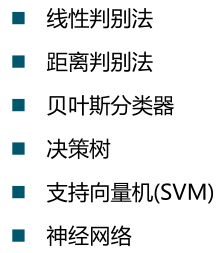线性判别法
简单来说就是用一些规定来寻找某一条直线，用直线划分学习集，然后根据待测点在直线的哪一边决定它的分类
如图，假如红色这条线是找出来的线，则要预测的那个红叉点为2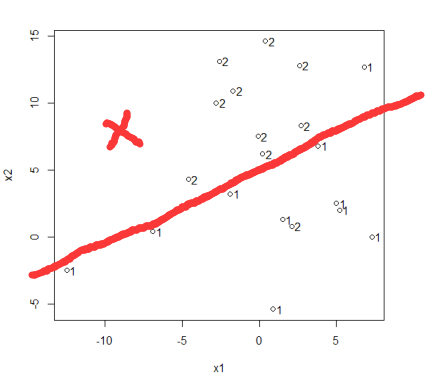距离判别法
简单来说就是预测某一个点的类别，分别计算这个点与各个样本点的距离（不是我们平常用的欧氏距离公式，而是马氏距离），这个点离哪个样本点最近，最近样本点是什么类别，他就是什么类别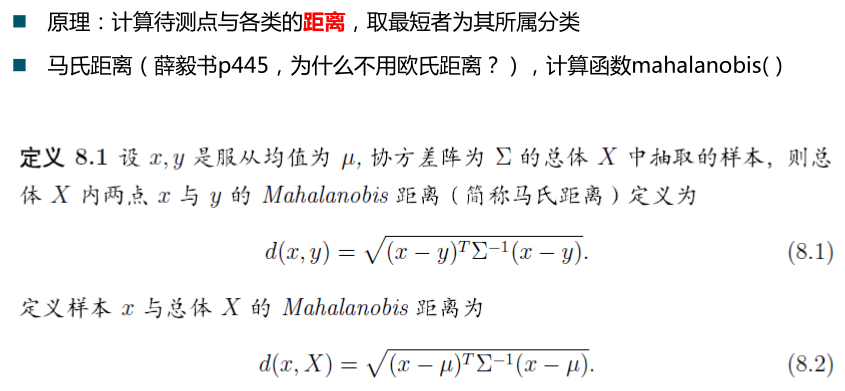最近邻算法（KNN）
简单来说，（一般K是我们定的一个数，一般K取奇数，这里我们k=5）我们要预测一个点的类别，计算该点与所有样本点的距离，取前k最小的距离，看一下这k个样本点类别最多的是什么类别，则预测点的样本类别与最多样本类别一致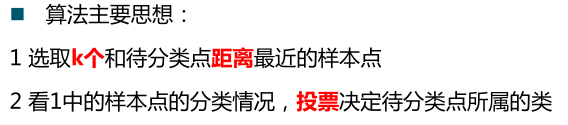如图，假如绿色叉叉点是我们要预测的点，距离最近的五个样本点类别分别是1,1,1,1,2，则预测的样本点分类为1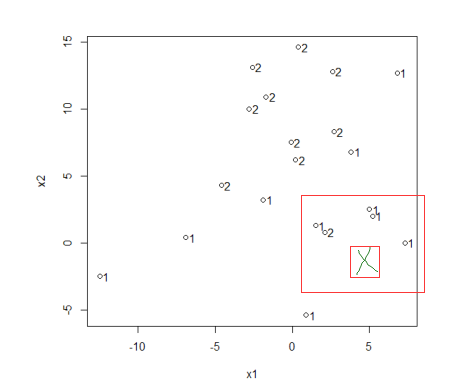贝叶斯分类器
在了解贝叶斯分类器之前我们来了解一下什么是先验概率和后验概率
先验概率
直观理解，所谓“先”，就是在事情之前，即在事情发生之前事情发生的概率。是根据以往经验和分析得到的概率。比如抛硬币，我们都认为正面朝上的概率是0.5，这就是一种先验概率，在抛硬币前，我们只有常识。这个时候事情还没发生，我们进行概率判断。所谓的先验概率是对事情发生可能性猜测的数学表示。
后验概率 事情已经发生了，事情发生可能有很多原因，判断事情发生时由哪个原因引起的概率。 比如今天你没去学校，原因有两个，可能是生病了，也可能是自行车坏了。然后上课时老师发现你没来。（这里是一个结果，就就是你没来学校这件事情已经发生了）老师叫学霸计算一下概率，分别是因为生病了没来学校的概率和自行车坏了没来学校的概率。 很显然，后验概率就是在事情发生后判断由哪一个原因引起的概率。这里的事情是你上学迟到，原因有生病了和自行车坏了。
贝叶斯公式
贝叶斯公式是由先验概率求后验概率的公式。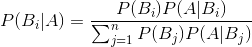在实际过程中后验概率一般是很难直接计算出来的，相反先验概率就容易多了。因此一般会利用先验概率来计算后验概率。这个时候贝叶斯公式就派上用场了。  从网上找到一个例子感觉说的比较好懂，以此来理解贝叶斯公式：  1）先验——根据若干年的统计（经验）或者气候（常识），某地方下雨的概率；  2）似然——下雨（果）的时候有乌云（因/证据/观察的数据）的概率，即已经有了果，对证据发生的可能性描述；  3）后验——根据天上有乌云（原因或者证据/观察数据），下雨（结果）的概率；  后验 ~ 先验*似然 ： 存在下雨的可能（先验），下雨之前会有乌云（似然）~ 通过现在有乌云推断下雨概率（后验）；  后验分布往往是基于先验分布和极大似然估计计算出来的。
贝叶斯垃圾邮件过滤器
问题是什么？问题是，给定一封邮件，判定它是否属于垃圾邮件。按照先例，我们还是用 D 来表示这封邮件，注意 D 由 N 个单词组成。我们用 h+ 来表示垃圾邮件，h- 表示正常邮件。问题可以形式化地描述为求：
P(h+|D) = P(h+) * P(D|h+) / P(D)
P(h-|D) = P(h-) * P(D|h-) / P(D)
其中 P(h+) 和 P(h-) 这两个先验概率都是很容易求出来的，只需要计算一个邮件库里面垃圾邮件和正常邮件的比例就行了。然而 P(D|h+) 却不容易求，因为 D 里面含有 N 个单词 d1, d2, d3, .. ，所以P(D|h+) = P(d1,d2,..,dn|h+) 。我们又一次遇到了数据稀疏性，为什么这么说呢？P(d1,d2,..,dn|h+) 就是说在垃圾邮件当中出现跟我们目前这封邮件一模一样的一封邮件的概率是多大！开玩笑，每封邮件都是不同的，世界上有无穷多封邮件。瞧，这就是数据稀疏性，因为可以肯定地说，你收集的训练数据库不管里面含了多少封邮件，也不可能找出一封跟目前这封一模一样的。结果呢？我们又该如何来计算 P(d1,d2,..,dn|h+) 呢？
我们将 P(d1,d2,..,dn|h+)  扩展为： P(d1|h+) * P(d2|d1, h+) * P(d3|d2,d1, h+) * .. 。熟悉这个式子吗？这里我们会使用一个更激进的假设，我们假设 di 与 di-1 是完全条件无关的，于是式子就简化为 P(d1|h+) * P(d2|h+) * P(d3|h+) * .. 。这个就是所谓的条件独立假设，也正是朴素贝叶斯方法的朴素之处。而计算 P(d1|h+) * P(d2|h+) * P(d3|h+) * .. 就太简单了，只要统计 di 这个单词在垃圾邮件中出现的频率即可。
朴素贝叶斯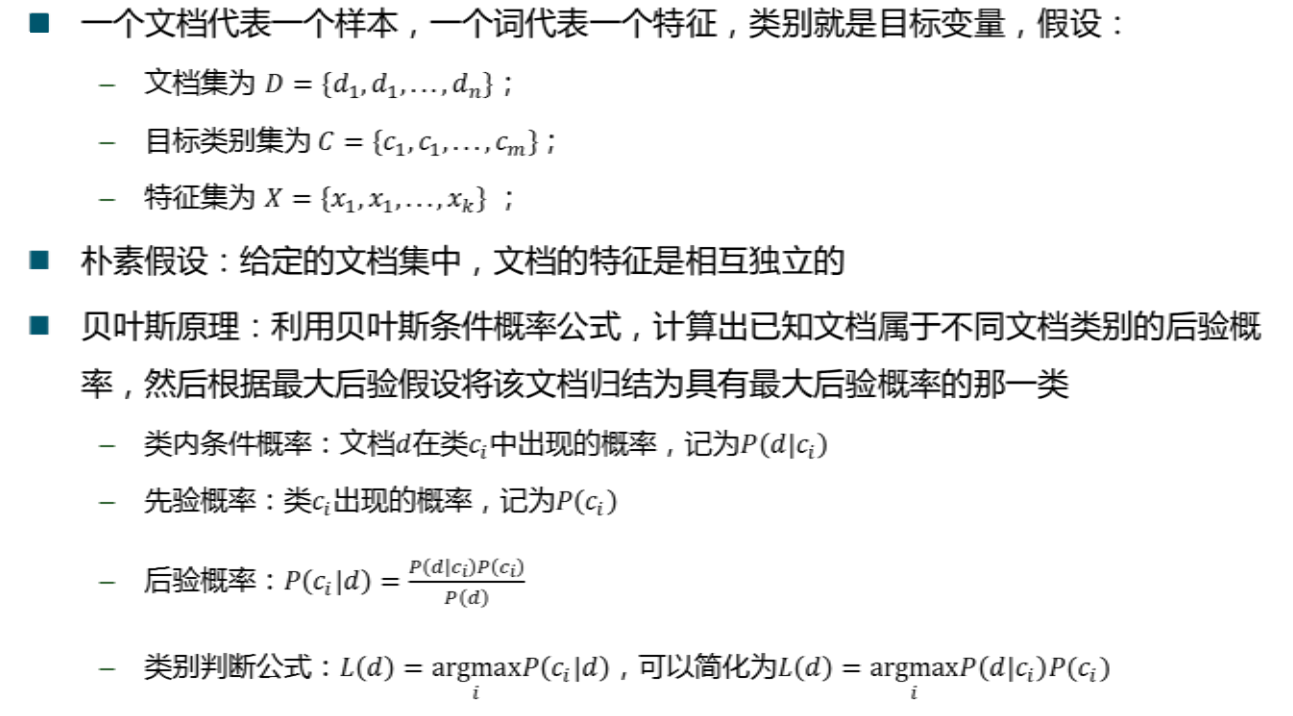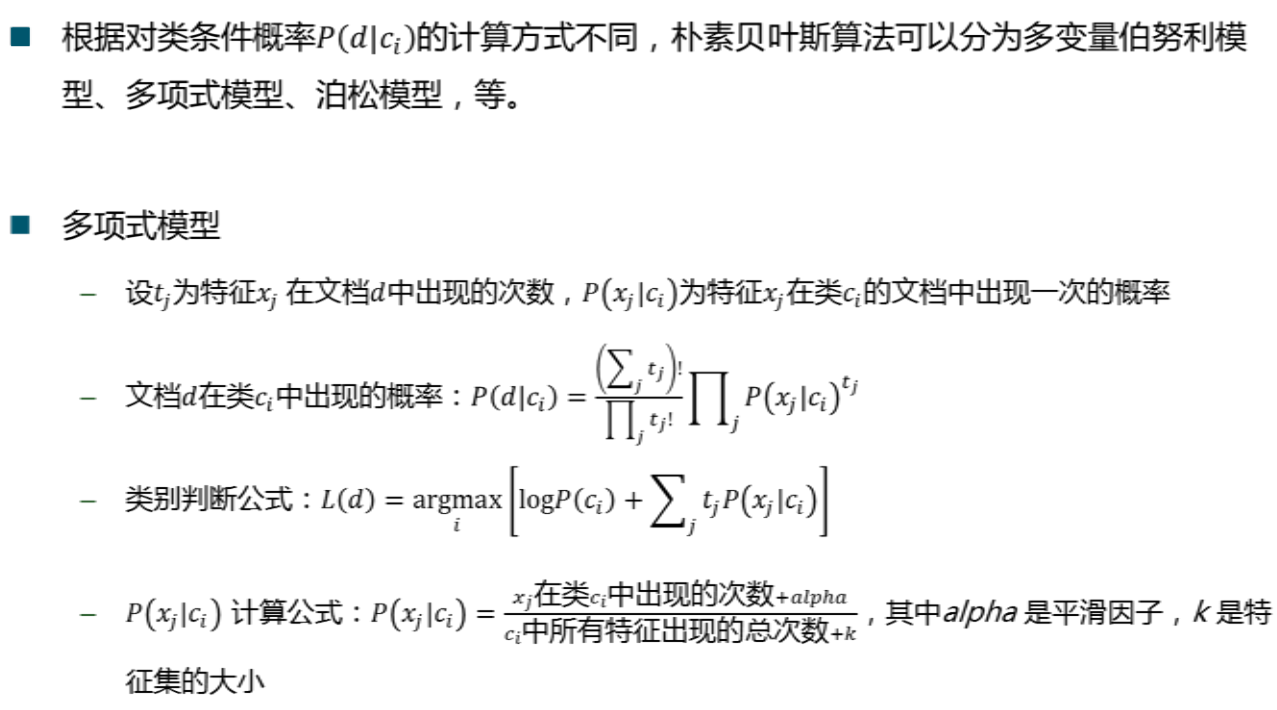贝叶斯信念网络
朴素贝叶斯考虑特征之间完全相符独立，但是现实中特征与特征都会有联系，贝叶斯信念网络用有向无环图表示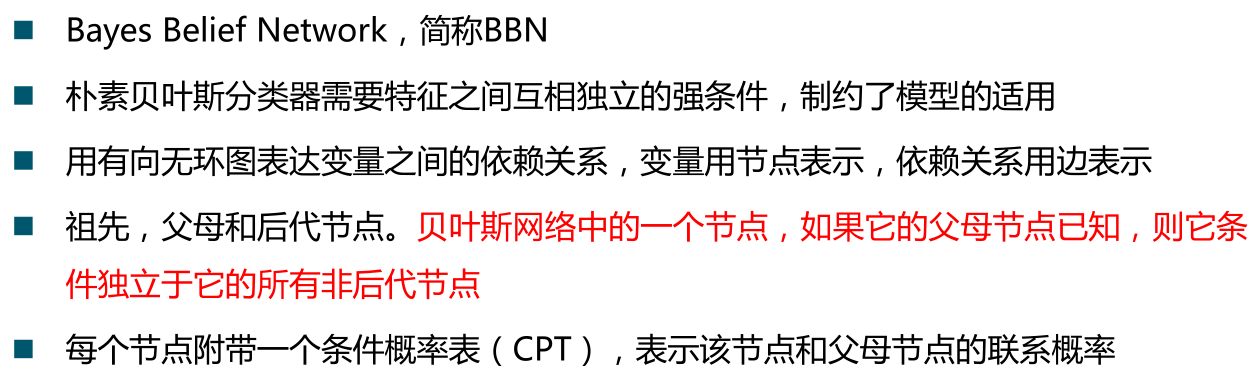建模步骤CPT计算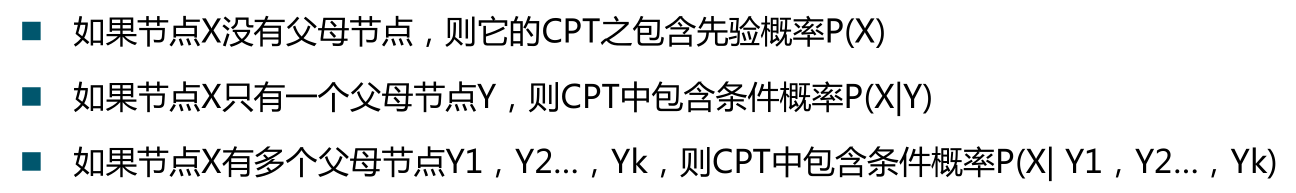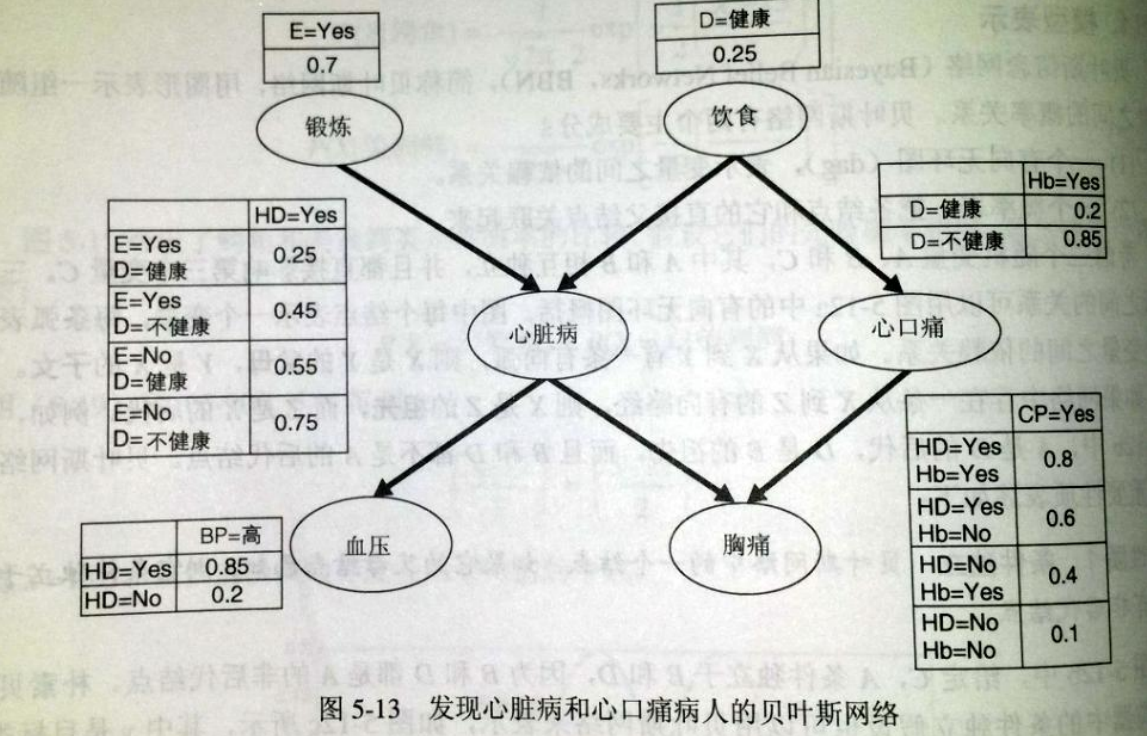展开全文分类 机器学习 距离判别法
• 线性判别分析（Linear Discriminant Analysis） 线性判别分析Linear Discriminant Analysis 线性分类器 判别式函数discriminant functions从判别式或后验概率到决策面 线性判别分析Linear ...
• 贝叶斯公式出发，得到了线性判别分析的公式，这里从另外一个角度来看线性判别分析，也就是常说的Fisher判别式。其实Fisher判别式就是线性判别分析（LDA），只是在讨论Fisher判别式的时候，更侧重于LDA的数据降维的...
• 线性判别分析（LDA）特别受欢迎，因为它既是分类器又是降维技术。二次判别分析（QDA）是LDA的变体，允许数据的非线性分离。最后，正则化判别分析（RDA）是LDAQDA之间的折衷。 本文主要关注LDA，并探讨其在理论...
• 线性判别分析（LDA）特别受欢迎，因为它既是分类器又是降维技术。二次判别分析（QDA）是LDA的变体，允许数据的非线性分离。最后，正则化判别分析（RDA）是LDAQDA之间的折衷。 本文主要关注LDA，并探讨其在理论...
• 一年前就研究过Fisher线性判别分析，到现在又忘得差不多了，在此特总结一下： 1、线性判别分析是统计学上的一种分析方法，用于在已知的分类之下遇到有新的样本时，选定一个判别标准，以判定如何将新样本放置于哪...类间离散矩阵 类内离散矩阵 LDA详解
• 转载地址：https://blog.csdn.net/daunxx/article/details/51881956https://blog.csdn.net/z962013489/article/details/79918758  LDA推广到多分类任务上 用来降维线性判别分析Linear Discriminant Analysis线性...
• 概率线性判别分析 论文引文 免责声明 通过经验贝叶斯估计参数。 该代码最初是为的人工智能（XAI）项目，因此它会将对于简单分类问题不必要的参数保留在内存中。 谢谢！ 特别感谢推动实现了相同的区别pip...Python
• 本文结构： 什么是 LDA PCA 区别 LDA 降维的计算过程 LDA 降维的例子 1. 什么是 LDA先说判别分析，Discriminant Analysis 就是根据研究...例如，在 KNN 中用的是距离判别，朴素贝叶斯分类用的是 Bayes 判别线性机器学习
• ## 线性判别分析 LDA

千次阅读 2016-06-20 14:59:10
Linear Discriminant Analysis 线性判别分析 LDALDA 降维
• ML之NB：基于news新闻文本数据集利用纯统计法、kNN、朴素贝叶斯(高斯/多元伯努利/多项式)、线性判别分析LDA、感知器等算法实现文本分类预测 目录 基于news新闻文本数据集利用纯统计法、kNN、朴素贝叶斯...
• Discriminant Analysis就是根据研究对象的各种特征值判别其类型归属问题的一种多变量统计分析方法。 根据判别标准不同，可以分为距离判别、Fisher判别、Bayes...本文要讲的线性判别分析就是用是Fisher判别式。 根据判lda算法原理
• 该方法结合了主成分分析（PCA）和线性判别分析（LDA）以减少高光谱数据的维数。 首先，将PCA用于一维降维，并获得非奇异的类内散布矩阵。 其次，将LDA应用于第二维降维，大大减少了计算量。 最后，将相关向量机模型...
• PCA、因子分析的区别： 聚类分析的关系： wiki中举得LDA对数据分为两类的例子 超平面 由此可以看出，LDA即是超平面上的分类。 Fisher’s Linear Discriminant Analysis(FDA) 代码：参考 ...
• 利用统计软件SPSS的逐步判别分析功能筛选出判别无烟煤、烟煤、褐煤的主要指标——氢含量氧含量,以该指标为变量建立贝叶斯逐步线性判别函数,并采用该函数分别对建模样本测试样本进行识别。识别结果显示:基于SPSS...
• 线性判别分析LDA为了最优分类，我们要计算后验概率P(G|X)P(G|X)。设fk(x)设f_k(x)是类G=kG=k中XX的类条件密度，而πk\pi_k是类kk的先验概率，贝叶斯定理给出 P(G=k|X=x)=fk(x)πk∑Kl=1fl(x)πlP(G=k|X=x)={f_k(x)...机器学习 统计学习基础 分类 数据降维
• 4. 实例  将3维空间上的球体样本点投影到二维上，W1相比W2能够获得更好的分离效果。   ... LDA既然叫做线性判别分析，应该具有一定的预测功能，比如新来一个样例x，如何确定其类别？LDA
• （1）高维特征的两类线性判别问题可以看作是把所有样本都投影到一个方向上，然后在这个一维样本空间中确定一个分类的阈值。过这个阈值点且与投影方向垂直的超平面就是两类的分类面。 （2）由（1），我们可知，所谓......